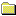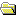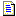Products Download Events Support Videos All Product Families ARM7, ARM9, and Cortex-M3 Products C16x, XC16x, and ST10 Products C251 and 80C251 Products Cx51 and 8051 Products Modified Anytime In the Last 7 Days In the Last 14 Days In the Last 30 Days In the Last 90 Days In the Last 6 Months

Technical Support

On-Line Manuals

Cx51 User's GuideCx51 IntroductionCompiling ProgramsLanguage ExtensionsPreprocessorAdvanced ProgrammingCustomization FilesStartup CodeVariable Initialization CodeXBANKING.A51Basic I/OMemory AllocationOptimizerOptimizer OptionsGeneral Optimizations8051-Specific OptimizationsGlobal Register ColoringSegment Naming ConventionsData ObjectsProgram ObjectsParameters and Local VariablesInterfacing C to AssemblerGlobal VariablesFunction ParametersPassing in MemoryPassing in RegistersFunction Return ValuesUsing the SRC DirectiveRegister UsageOverlaying SegmentsSmall Model ExampleCompact Model ExampleLarge Model ExampleInterfacing C programs to PL/M-51Data Storage FormatsBit Variables1-Byte Scalars2-Byte Scalars4-Byte ScalarsGeneric and Far PointersFloating-point NumbersFloating-point ErrorsAbsolute Memory LocationsAbsolute Access MacrosLinker Location ControlsThe _at_ KeywordDebugging8051 DerivativesError MessagesLibrary ReferenceAppendix

# <!-- var kmNextPage = "c51_ap_floaterror.htm"; var kmPrevPage = "c51_ap_genericptr.htm"; var kmNextPageTitle = "Floating-point Errors"; var kmPrevPageTitle = "Generic and Far Pointers"; var kmBreadCrumbs = "<a href=\"default.htm\">Home</a> &raquo; <a href=\"c51_advanced.htm\">Advanced Programming</a> &raquo; <a href=\"c51_ap_datastorage.htm\">Data Storage Formats</a> &raquo; Floating-point Numbers"; kmSetupPaging (); kmNavButtons(); //--> Floating-point Numbers

Scalars of type float are stored using four bytes (32-bits). The format used follows the IEEE-754 standard.

A floating-point number is expressed as the product of two parts: the mantissa and a power of two. For example:

±mantissa × 2exponent

The mantissa represents the actual binary digits of the floating-point number.

The power of two is represented by the exponent. The stored form of the exponent is an 8-bit value from 0 to 255. The actual value of the exponent is calculated by subtracting 127 from the stored value (0 to 255) giving a range of –127 to +128.

The mantissa is a 24-bit value (representing about seven decimal digits) whose most significant bit (MSB) is always 1 and is, therefore, not stored. There is also a sign bit that indicates whether the floating-point number is positive or negative.

Floating-point numbers are stored on byte boundaries in the following format:

Contents `SEEE EEEE` `EMMM MMMM` `MMMM MMMM` `MMMM MMMM`

Where

 S represents the sign bit where 1 is negative and 0 is positive. E is the exponent with an offset of 127. M is the 24-bit mantissa (stored in 23 bits).

Zero is a special value denoted with an exponent field of 0 and a mantissa of 0.

Using the above format, the floating-point number -12.5 is stored as a hexadecimal value of 0xC1480000. In memory, this value appears as follows:

Contents 0xC1 0x48 0x00 0x00

It is fairly simple to convert floating-point numbers to and from their hexadecimal storage equivalents. The following example demonstrates how this is done for the value -12.5 shown above.

The floating-point storage representation is not an intuitive format. To convert this to a floating-point number, the bits must be separated as specified in the floating-point number storage format table shown above. For example:

Format `SEEEEEEE` `EMMMMMMM` `MMMMMMMM` `MMMMMMMM`
Binary `11000001` `01001000` `00000000` `00000000`
Hex `C1` `48` `00` `00`

From this illustration, you can determine the following:

• The sign bit is 1, indicating a negative number.
• The exponent value is 10000010 binary or 130 decimal. Subtracting 127 from 130 leaves 3, which is the actual exponent.
• The mantissa appears as the following binary number:
```10010000000000000000000
```

There is an understood binary point at the left of the mantissa that is always preceded by a 1. This digit is omitted from the stored form of the floating-point number. Adding 1 and the binary point to the beginning of the mantissa gives the following value:

```1.10010000000000000000000
```

To adjust the mantissa for the exponent, move the decimal point to the left for negative exponent values or right for positive exponent values. Since the exponent is three, the mantissa is adjusted as follows:

```1100.10000000000000000000
```

The result is a binary floating-point number. Binary digits to the left of the decimal point represent the power of two corresponding to their position. For example, 1100 represents (1 × 23) + (1 × 22) + (0 × 21) + (0 × 20), which is 12.

Binary digits to the right of the decimal point also represent the power of two corresponding to their position. However, the powers are negative. For example, .100... represents (1 × 2-1) + (0 × 2-2) + (0 × 2-3) + ... which equals .5.

The sum of these values is 12.5. Because the sign bit was set, this number should be negative.

So, the hexadecimal value 0xC1480000 is -12.5.##### Important information

This site uses cookies to store information on your computer. By continuing to use our site, you consent to our cookies.